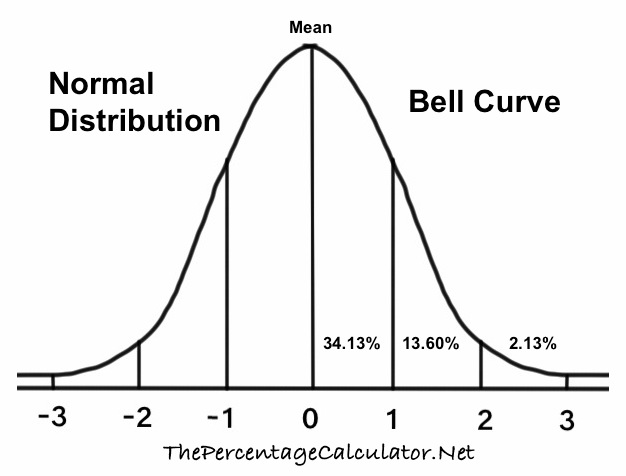Z Score 2.251We looked up the Z Score for 2.251 in our Normal Distribution Tables with Z Scores so you don't have to!

First, note that a Z Score of 2.251 means that your statistic is 2.251 standard deviation to the right of the mean on a bell curve. Here is a Bell Curve so you can visualize where 2.251 is on a bell curve.We used three different distribution tables, and we will give you the 2.251 Z Score probability, percentile, and explanations for all three.

Cumulative from mean
This gives you the probability of the area from the mean to your Z Score. The cumulative from-mean probability and percentile for a 2.251 Z score is displayed here:

0.487807231311167
= 48.7807%

Cumulative
This gives you the probability of the area below the Z Score. The cumulative probability and percentile for a 2.251 Z score is displayed here:

0.987807231311167
= 98.7807%

Complementary cumulative
This gives you the probability of the area above the Z Score. The complementary cumulative probability and percentile for a 2.251 Z Score is displayed here:

0.012192768688833
= 1.2193%

Z Score Table Lookup
Here you can submit Z Scores between -3.999 and 3.999 for us to look up in our Normal Distribution Tables.

Z Score 2.252
Here is the next Z Score we looked up in our Normal Distribution Tables.

The percentiles on this page are rounded to the nearest 4 decimals.